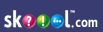Solubility of Substances Generix Content - Solubility of Substances
"Solubility of Substances" question number distribution across years
loading...
Chemistry index on skoool nigeriaWAEC questions for "Chemistry :: Solubility of Substances"
Q1

A solution that contains as much solute as it can dissolve at a given temperature is said to be

A

concentrated

B

saturated

C

supersaturated

D

unsaturated

E
Q2

Which of the following statements is correct? The solubility of

A

gases increases with increase in temperature

B

gases decreases with increase in temperature

C

most solid solutes decreases with increase in temperature

D

Most solid solutes is constant

E
Q3

Consider the reaction represented by the equation below: KOH(aq) + HCl(aq) → KCl(aq) + H2O(l). What volume of 0.25mol dm-3 KOH would be required to completely neutrlize 40cm3 of 0.10mol dm-3 HCl?

A

40cm3

B

32cm3

C

24cm3

D

16cm3

E
Q4

When 100cm3 of a saturate solution of KClO3 at 40°C is evaporated, 14g of the salt is recovered. What is the solubility of KClO3 at 40°C? [KClO3 = 122.5]

A

11.42mol dm-3

B

8.80mol dm-3

C

1.14mol dm-3

D

0.88mol dm-3

E
Q5

The high solubility of ethanol in water is due its

A

low boiling point

B

low freezing point

C

covalent nature

D

hydrogen bond

E
Q6

25cm3 of 0.80mol dm-3 hydrolic acid neutralizaed 20cm3 of sodium hydroxide solution. What is the concentration of sodium hydroxide in mol dm-3 ? NaOH(aq) + HCl(aq) → NaCl(aq) + H2O(l)

A

0.08

B

0.10

C

80

D

1.00

E
Q7

When 50cm3 of a saturated solution of KNO3 at 25°C was evaporated to dryness, 10g of dry salt was obtained. What is the solubility of KNO3 at 25°C? [KNO3 = 101]

A

0.10mol dm-3

B

2.0mol dm-3

C

5.0mol dm-3

D

10.0mol dm-3

E
Q8

Which of the following compoundsss dissolves readily in water?

A

BaSO4

B

CuCO3

C

NH4Cl

D

AgCl

E
Q9

A solution of sodium hydroxide containing 6.0g in 250cm3 of solution has a concentration of

[molar mass on NaOH = 40g mol-1]

A

0.04moldm-3

B

0.60mol dm-3

C

0.96mol dm-3

D

0.15mol dm-3

E
Q10

Which of the following statements about X and Z is correct?

A

X is the more soluble solid at room temperature

B

X and Z are soluble at 0ºC

C

0.20moldm-3 solution of Z at 10°C is unsaturated

D

Z is more soluble than X at room temperature below 20ºC

E
Q11

What is the solubility of Z at 50°C?

A

0.20mol dm-3

B

0.25mol dm-3

C

0.35mol dm-3

D

0.50mol dm-3

E
Q12

If 10cm3 of distilled water is added to 10cm3 of an aqueous  salt solution, the concentration of the solution

A

increases

B

decreases

C

remains constant

D

doubles

E
Q13

What type of reaction is involved when wood shaving produce a brown gas with concentrated HNO3?

A

Redox reaction

B

Destructive distillation

C

Dehydration

D

Neutralization

E
Q14

If 20cm3 of distilled water is added to 80cm3 of 0.50moldm3 hydrochloric acid, the concentration of the acid will change to

A

0.20mol dm-3

B

0.40mol dm-3

C

2.00mol dm-3

D

5.00mol dm-3

E
Q15

Consider the reaction represented by the following equation: H2(g) + I2(g) → 2HI(g). The number of effective collision of molecules of reactants decreases as

A

the temperature is raised

B

the pressure is increased

C

the volume of the system is increased

D

a catalyst is introduced into the system

E
Q16

The loss of molecules of water of crystallization to the atmosphere by some crystalline salt is known as

A

efflorescence

B

effervescence

C

phosphorescence

D

flourescence

E

deliquescence

Q17

Ammonia gas is suitable for performing the 'Fountain' experiment because the gas is

A

Colourless

B

a cooling agent

C

alkaline to litmus

D

a reducing agent

E

very soluble in water

Q18

When a crystal was added to its solution, it did not dissolve and the solution remained unchanged, showing that the solution was

A

Concentrated

B

Supersaturated

C

unsaturated

D

colloidal

E

saturated

Q19

Which of the following compound crystallize wthout water of crystallization?

A

Na2CO3

B

CuSO4

C

MgSO4

D

NaCl

E

FeSO4

Q20

What is the concentration in mol dm-3, of a solution containing 0.10g of sodium hydroxide per 50cm3 of solution? (NaOH = 40)

A

0.05

B

0.1

C

0.5

D

2

E

5

Q21

50cm3 of saturated solution of potassium trioxonitrate (V) at 40contained 5.05g of the salt what is the solubility of potassium trioxonitrate (V) at 400 C ? (KNO3 = 101)

A

1.0 mol dm-3

B

1.5 mol dm-3

C

2.0 mol dm-3

D

2.5 mol dm-3

E

5.0 mol dm-3